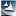# 安全通论（3）：攻防篇之“非盲对抗”之“石头剪刀布”精选

------攻防篇之“非盲对抗”之“石头剪刀布”

（一）前言

（二）信道建模

Pr(X=0)=p，即，甲出“剪刀”的概率；

Pr(X=1)=q，即，甲出“石头”的概率；

Pr(X=2)=1-p-q，即，甲出“布”的概率。这里0<p,q,p+q<1

Pr(Y=0)=r，即，乙出“剪刀”的概率；

Pr(Y=1)=s，即，乙出“石头”的概率；

Pr(Y=2)=1-r-s，即，乙出“布”的概率。这里0<r,s,r+s<1

Pr(X=0,Y=0)=a，即，甲出“剪刀”，乙出“剪刀”的概率；

Pr(X=0,Y=1)=b，即，甲出“剪刀”，乙出“石头”的概率；

Pr(X=0,Y=2)=1-a-b，即，甲出“剪刀”，乙出“布”的概率。这里0<a,b,a+b<1

Pr(X=1,Y=0)=e，即，甲出“石头”，乙出“剪刀”的概率；

Pr(X=1,Y=1)=f，即，甲出“石头”，乙出“石头”的概率；

Pr(X=1,Y=2)=1-e-f，即，甲出“石头”，乙出“布”的概率。这里0<e,f,e+f<1

Pr(X=2,Y=0)=g，即，甲出“布”，乙出“剪刀”的概率；

Pr(X=2,Y=1)=h，即，甲出“布”，乙出“石头”的概率；

Pr(X=2,Y=2)=1-g-h，即，甲出“布”，乙出“布”的概率。这里0<g,h,g+h<1

1）针对甲方来说，对任意k/nC，都一定有某种技巧（对应于仙农编码），使得，在nC次游戏中，甲方能够胜乙方k次；如果在某m次游戏中，甲方已经胜出乙方u次，那么，一定有umC。这里C是“甲方信道”的容量。

2）针对乙方来说，对任意k/nD，都一定有某种技巧（对应于仙农编码），使得，在nD次游戏中，乙方能够胜甲方k次；如果在某m次游戏中，乙方已经胜出甲方u次，那么，一定有umD。这里D是“乙方信道”的容量。

3）如果C<D，那么，整体上甲方会输；如果C>D，那么，整体上甲方会赢；如果C=D，那么，甲乙双方势均力敌。

（三）巧胜策略

3.1：两个傻瓜之间的游戏

3.2 一个傻瓜与一个智者之间的游戏

3.3 两个智者之间的游戏

（四）简化版本

0<Pr(X=x)=px<1x=0,1,2p0+p1+p2=1

0<Pr(Y=y)=qy<1y=0,1,2q0+q1+q2=1

0<Pr(X=x,Y=y)=txy<1x,y=0,1,20≤x,y≤2txy=1

px=0≤y≤2txyx=0,1,2

qy=0≤x≤2txyy=0,1,2

“石头剪刀布”游戏的输赢规则是：若X=xY=y，那么，甲（X）赢的充分必要条件是：(y-x)mod3=2

XF）的信道容量=MaxX[I(X,F)]=MaxX[I(X,(Y-2)mod3)]=MaxX[I(X,Y)]=MaxX[txylog(txy/(pxqy))]，这里的最大值，是针对所有可能的txypx而取的，所以，它实际上是q0q1q2的函数。

YG）的信道容量=MaxY[I(Y,G)]=MaxY[I(Y,(X-2)mod3)]=MaxY[I(X,Y)]=MaxY[txylog(txy/(pxqy))]，这里的最大值，是针对所有可能的txyqy而取的，所以，它实际上是p0p1p2的函数。

（五）结束语

“攻防”是安全的核心，所以，在建立“安全通论”的过程中，多花一些精力去深入研究“攻防”也是值得的。

中，我们研究了“安全通论”的盲对抗问题，本文研究的“石头剪刀布”游戏则是一种“非盲对抗”，但由于它的普及率极高（几千年来，全世界每个人在童年时代几乎都玩过），所以，我们以单独一篇论文的形式来研究它。有关其它一些有代表性的“非盲对抗”，我们将在随后的文章中研究。安全通论（3），攻防篇之”非盲对抗“之“石头剪刀布”.docx

杨义先，钮心忻，安全通论（1）之“经络篇”，见杨义先的科学网实名博客（

杨义先，钮心忻，安全通论（2）：攻防篇之“盲对抗”，见杨义先的科学网实名博客，（http://blog.sciencenet.cn/blog-453322-947304.html

Thomas M. Cover Joy A. Thomas著，信息论基础，机械工业出版社出版，200711月，北京。阮吉寿，张华译；沈世镒审校。

Shu LinDaniel J. Costello,Jr.著，差错控制码，机械工业出版社，20076月，北京。晏坚，何元智，潘亚汉等译。

https://wap.sciencenet.cn/blog-453322-948089.html

## 相关博文

GMT+8, 2022-5-28 21:59